# Code Generation for a Deep Learning Simulink Model to Classify ECG Signals

This example demonstrates how you can use powerful signal processing techniques and Convolutional Neural Networks together to classify ECG signals. We will also showcase how CUDA® code can be generated from the Simulink® model. This example uses the pretrained CNN network from the Classify Time Series Using Wavelet Analysis and Deep Learning example of the Wavelet Toolbox™ to classify ECG signals based on images from the CWT of the time series data. For information on training, see Classify Time Series Using Wavelet Analysis and Deep Learning (Wavelet Toolbox).

This example illustrates the following concepts:

• Model the classification application in Simulink. Use `MATLAB Function` blocks to perform preprocessing and wavelet transforms of the ECG data. Use the `Image Classifier` block from the Deep Learning Toolbox™ for loading the pretrained network and performing the classification of the ECG data.

• Configure the model for code generation.

• Generate a CUDA executable for the Simulink model.

### Verify GPU Environment

To verify that the compilers and libraries necessary for running this example are set up correctly, use the `coder.checkGpuInstall` function.

```envCfg = coder.gpuEnvConfig('host'); envCfg.DeepLibTarget = 'cudnn'; envCfg.DeepCodegen = 1; envCfg.Quiet = 1; coder.checkGpuInstall(envCfg); ```

### ECG Data Description

This example uses ECG data from PhysioNet database. It contains data from three groups of people:

1. Persons with cardiac arrhythmia (ARR)

2. Persons with congestive heart failure (CHF)

3. Persons with normal sinus rhythms (NSR)

It includes 96 recordings from persons with ARR, 30 recordings from persons with CHF, and 36 recordings from persons with NSR. The `ecg_signals` MAT-file contains the test ECG data in time series format. The image classifier in this example distinguishes between ARR, CHF, and NSR.

### Algorithmic Workflow

The block diagram for the algorithmic workflow of the Simulink model is shown.### ECG Deep Learning Simulink Model

The Simulink model for classifying the ECG signals is shown. When the model runs, the `Video Viewer` block displays the classified ECG signal.

```open_system('ecg_dl_cwt'); ```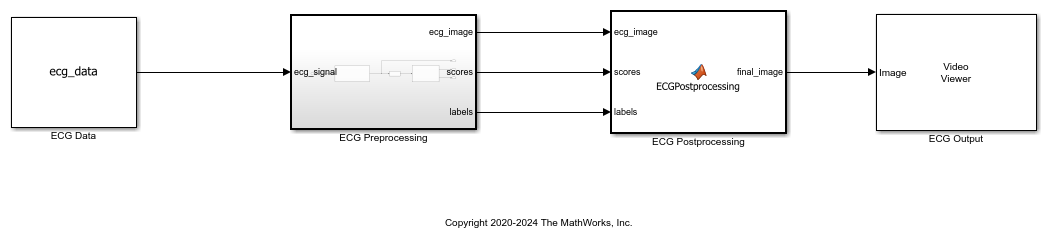### ECG Preprocessing Subsystem

The `ECG Preprocessing` subsystem contains a `MATLAB Function` block that performs CWT to obtain scalogram of the ECG signal and then processes the scalogram to obtain an image and an `Image Classifier` block that loads the pretrained network from `trainedNet.mat` and performs prediction for image classification based on SqueezeNet deep learning CNN.

```open_system('ecg_dl_cwt/ECG Preprocessing'); ```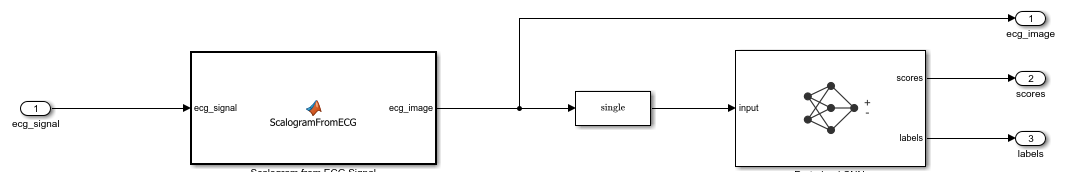The `ScalogramFromECG` function block defines a function called `ecg_to_scalogram` that:

• Uses 65536 samples of double-precision ECG data as input.

• Create time frequency representation from the ECG data by applying Wavelet transform.

• Obtain scalogram from the wavelet coefficients.

• Convert the scalogram to image of size (227x227x3).

The function signature of `ecg_to_scalogram` is shown.

```type ecg_to_scalogram ```
```function ecg_image = ecg_to_scalogram(ecg_signal) % Copyright 2020 The MathWorks, Inc. persistent jetdata; if(isempty(jetdata)) jetdata = colourmap(128,'single'); end % Obtain wavelet coefficients from ECG signal cfs = cwt_ecg(ecg_signal); % Obtain scalogram from wavelet coefficients image = ind2rgb(im2uint8(rescale(cfs)),jetdata); ecg_image = im2uint8(imresize(image,[227,227])); end ```

### ECG Postprocessing

The `ECG Postprocessing` MATLAB function block defines the `label_prob_image` function that finds the label for the scalogram image based on the highest score from the scores outputed by the image classifier. It outputs the scalogram image with the label and confidence printed on it.

```type label_prob_image ```
```function final_image = label_prob_image(ecg_image, scores, labels) % Copyright 2020 The MathWorks, Inc. scores = double(scores); % Obtain maximum confidence [prob,index] = max(scores); confidence = prob*100; % Obtain label corresponding to maximum confidence label = erase(char(labels(index)),'_label'); text = cell(2,1); text{1} = ['Classification: ' label]; text{2} = ['Confidence: ' sprintf('%0.2f',confidence) '%']; position = [135 20 0 0; 130 40 0 0]; final_image = insertObjectAnnotation(ecg_image,'rectangle',position,text,'TextBoxOpacity',0.9,'FontSize',9); end ```

### Run the Simulation

Open Configuration Parameters dialog box.

In Simulation Target pane, select GPU acceleration. In the Deep Learning group, select the target library as cuDNN.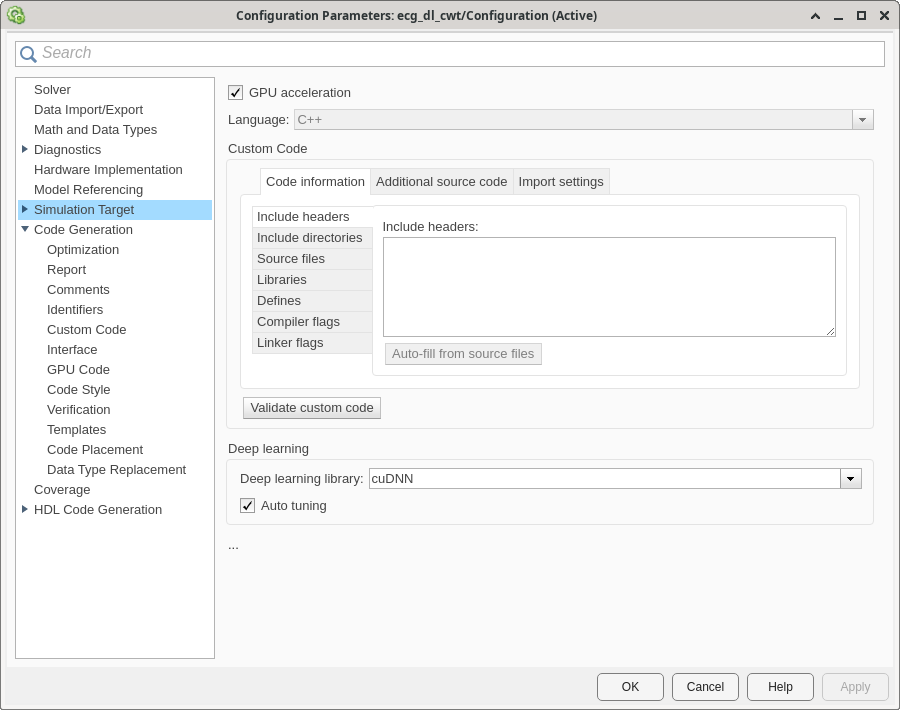To verify the algorithm and display the labels and confidence score of the test ECG signal loaded in the workspace, run the simulation.

```set_param('ecg_dl_cwt', 'SimulationMode', 'Normal'); sim('ecg_dl_cwt'); ```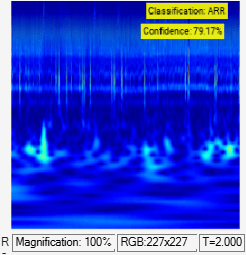### Generate and Build the Simulink Model

In Code Generation pane, select the Language as C++ and enable Generate GPU code.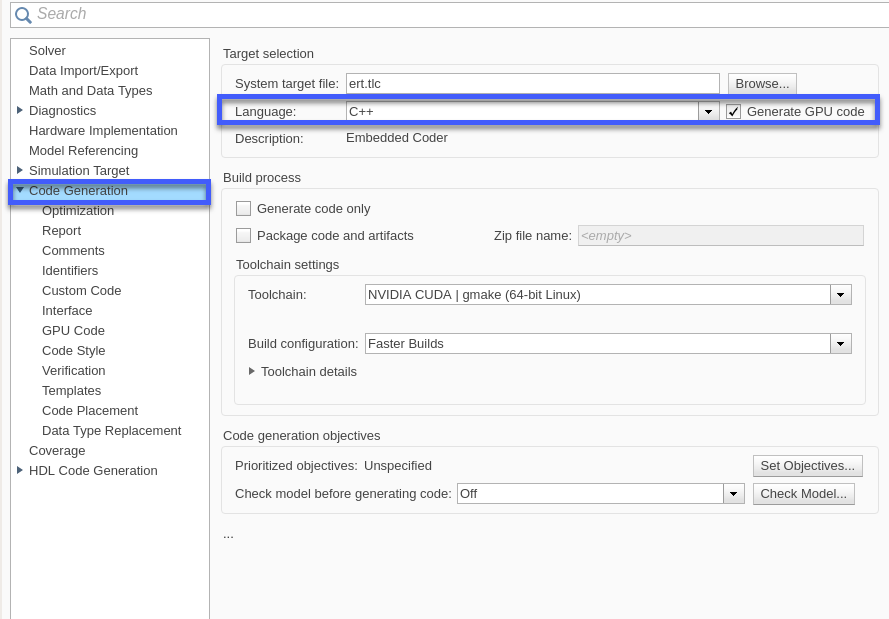Open Code Generation > GPU Code pane. In the subcategory Libraries, enable cuBLAS, cuSOLVER and cuFFT.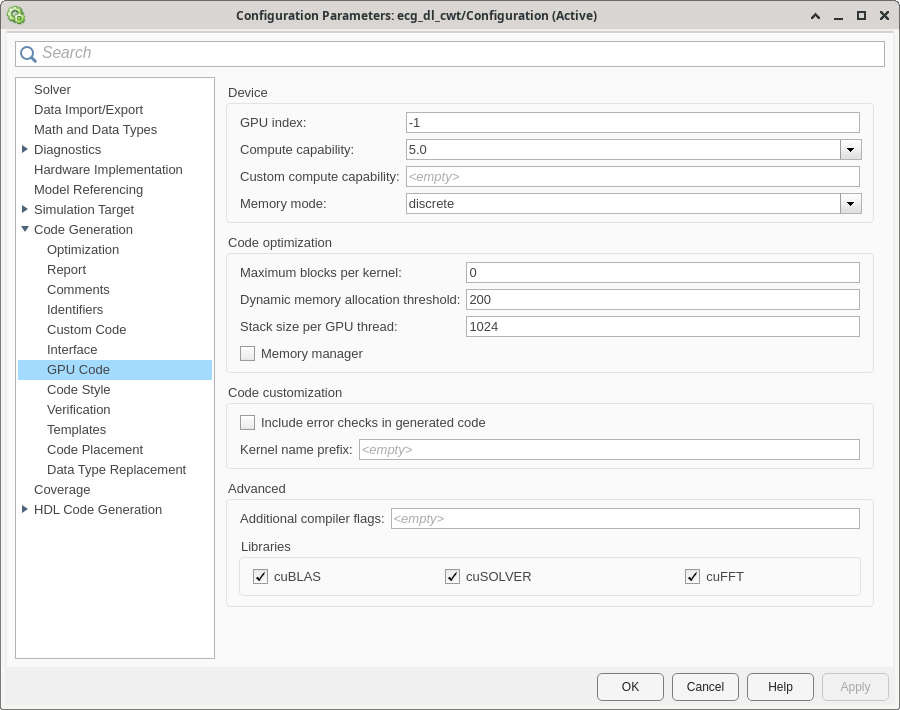Generate and build the Simulink model on the host GPU by using the `slbuild` command. The code generator places the files in a build folder, a subfolder named `ecg_dl_cwt_ert_rtw` under your current working folder.

```status = evalc("slbuild('ecg_dl_cwt')"); ```### Generated CUDA® Code

The subfolder named `ecg_dl_cwt_ert_rtw` contains the generated C++ codes corresponding to the different blocks in the Simulink model and the specific operations being performed in those blocks. For example, the file `trainedNet0_ecg_dl_cwt0.h` contains the C++ class which contains certain attributes such as `numLayers` and member functions such as `getBatchSize()`, `predict()`. This class represents the pretrained `SqueezeNet` which has been loaded in the Simulink model.### Cleanup

```close_system('ecg_dl_cwt/ECG Preprocessing'); close_system('ecg_dl_cwt'); ```